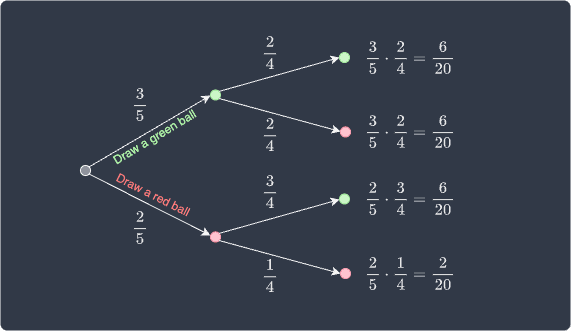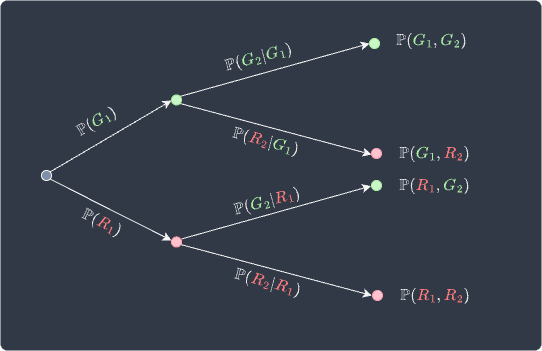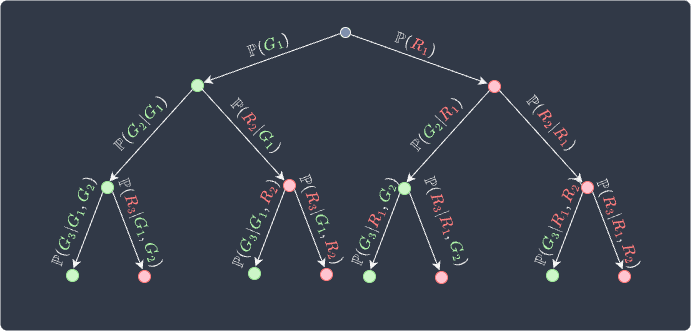search
Search
Map of Data Science
Guest 0reps
exit_to_appLog out
Map of data science
Thanks for the thanks!
close
Cancel
Post
account_circle
Profile
exit_to_app
Sign out
search
keyboard_voice
close
Searching Tips
Search for a recipe:
"Creating a table in MySQL"
Search for an API documentation: "@append"
Search for code: "!dataframe"
Apply a tag filter: "#python"
Useful Shortcuts
/ to open search panel
Esc to close search panel
to navigate between search results
d to clear all current filters
Enter to expand content previewDoc SearchCode Search BetaSORRY NOTHING FOUND!
mic
Start speaking...Voice search is only supported in Safari and Chrome.
Shrink
Navigate to
A
A
brightness_medium
share
arrow_backShareTwitterFacebook
check_circle
Mark as learned
thumb_up
0
thumb_down
0
chat_bubble_outline
0
auto_stories new
settings

# Comprehensive Guide on Conditional Probability

Probability and Statistics
chevron_right
Probability Theory
schedule Nov 1, 2022
Last updated
local_offer Probability and Statistics
Tags
map
Check out the interactive map of data science
Example.

# Drawing a probability tree diagram

Suppose we have a bag containing $5$ balls of which $3$ are green and $2$ are red. We randomly draw two balls in succession without replacement. What is the probability of each outcome?

Solution. The probability of each outcome can be summarized using a probability tree diagram:Here, each branch of the tree represents an outcome of our experiment and has an associated probability.

Let's now define the following events:

• event $\color{green}G_1$ - selecting a green ball in the first draw.

• event $\color{green}G_2$ - selecting a green ball in the second draw.

• event $\color{red}R_1$ - selecting a red ball in the first draw.

• event $\color{red}R_2$ - selecting a red ball in the second draw.

The probability that we first draw a green ball is given by:

$$\mathbb{P}({\color{green}G_1}) =\frac{3}{5}$$

The probability of the outcome of the second draw is affected by what ball is drawn first. This means that events associated with the second draw can be expressed using the so-called conditional probability. For instance, the probability that we draw a green ball given that we drew a red ball first is:

$$\mathbb{P}( {\color{green}G_2}| {\color{red}R_1}) =\frac{3}{4}$$

Here, the symbol $|$ is read as "given that". A probability of an event given the occurrence of another event is called conditional probability.

The probabilities on the right side of the tree diagram represent joint probabilities. For instance, the probability that we get a red ball and then a green ball is computed by:

\begin{align*} \mathbb{P}( {\color{red}R_1} ,{\color{green}G_2}) &= \mathbb{P}({\color{red}R_1})\cdot\mathbb{P}( {\color{green}G_2}| {\color{red}R_1})\\&= \frac{2}{5}\cdot\frac{3}{4} = \frac{6}{20} \end{align*}

In general, the probability tree diagram looks like the following:The joint probabilities at the leaves can be computed by multiplying the probabilities of the associated branches:

\begin{align*} \mathbb{P}( {\color{green}G_1} ,{\color{green}G_2}) &= \mathbb{P}({\color{green}G_2}\vert {\color{green}G_1})\cdot \mathbb{P}({\color{green}G_1})\\ \mathbb{P}( {\color{green}G_1} ,{\color{red}R_2}) &= \mathbb{P}({\color{red}R_2}\vert {\color{green}G_1})\cdot \mathbb{P}({\color{green}G_1})\\ \mathbb{P}( {\color{red}R_1} ,{\color{green}G_2}) &= \mathbb{P}({\color{green}G_2}\vert {\color{red}R_1})\cdot \mathbb{P}({\color{red}R_1})\\ \mathbb{P}( {\color{red}R_1} ,{\color{red}R_2}) &= \mathbb{P}({\color{red}R_2}\vert {\color{red}R_1})\cdot \mathbb{P}({\color{red}R_1}) \end{align*}

Notice how there is a pattern here. In general, the joint probability for any two events $A$ and $B$ can be expressed as:

$$\mathbb{P}(A,B)=\mathbb{P}(B|A)\cdot\mathbb{P}(A)$$

Rearranging this to make the conditional probability $\mathbb{P}(B|A)$ the subject:

$$\mathbb{P}(B|A)=\frac{\mathbb{P}(A,B)}{\mathbb{P}(A)}$$

We've managed to derive the general formula for the conditional probability of event $B$ given event $A$.

Definition.

# Conditional probability

The conditional probability of an event $B$ given that an event $A$ has occurred is:

$$\mathbb{P}(B|A)=\frac{\mathbb{P}(A,B)}{\mathbb{P}(A)}$$

Where:

• $\mathbb{P}(B|A)$ is read as the "probability of $B$ given $A$".

• $\mathbb{P}(A,B)$ is the probability that both $A$ and $B$ occur.

In set notation, the formula for conditional probability is written as:

$$\mathbb{P}(B|A)=\frac{\mathbb{P}(A\cap{B})}{\mathbb{P}(A)}$$

Where $\cap$ is the symbol for intersection.

Let's now go through some example questions about conditional probabilities!

Example.

## Rolling a dice

Suppose we roll a fair dice once. What is the probability that we roll a 3 given that the outcome is odd?

Solution. Let's define the following two events:

• event $A$ - we roll a $3$.

• event $B$ - we roll an odd number $\{1,3,5\}$.

The probability of rolling a $3$ given that the outcome is odd is:

\begin{align*} \mathbb{P}(A\vert{B})&= \frac{\mathbb{P}(A,B)}{\mathbb{P}(B)}\\ &=\frac{\mathbb{P}(A)}{\mathbb{P}(B)}\\ &=\frac{1/6}{3/6}\\ &=\frac{1}{3} \end{align*}

Here, $\mathbb{P}(A,B)=\mathbb{P}(A)$ in this case because the probability that the outcome is a $3$ and an odd number is equal to the probability that the outcome is $3$.

Example.

# Defective products and complaints

The probability that a product is defective is $0.5$. The probability that a product is defective and a customer complains is $0.2$. What is the probability that a customer complains given that the product is defective?

Solution. We first define the following events:

• event $A$ - customer complains.

• event $B$ - product is defective.

The probability that a customer complains given that the product is defective is:

$$\mathbb{P}(A|B) =\frac{\mathbb{P}(A,B)}{\mathbb{P}(B)} =\frac{0.2}{0.5} =0.4$$

Definition.

# Conditional probability of 3 events

If $A$, $B$ and $C$ are events, then the conditional probability of $C$ given $A$ and $B$ is:

$$P(C|A,B)= \frac{\mathbb{P}(A,B,C)} {\mathbb{P}(A)\cdot\mathbb{P}(B\vert{A})}$$

Intuition. Suppose we have a bag containing $7$ balls, of which $3$ are green and $4$ are red. We draw $3$ balls from this bag successively at random without replacement.

Let's define the following events:

• $\color{green}G_1$, $\color{green}G_2$ and $\color{green}G_3$ are the events of selecting a green ball on the 1st, 2nd and 3rd draw respectively.

• $\color{red}R_1$, $\color{red}R_2$ and $\color{red}R_3$ are the events of selecting a red ball on the 1st, 2nd and 3rd draw respectively.

Just as we did before, we can draw a probability tree diagram to express all the probabilities of each possible scenario:The product of the three probabilities of any branch gives us the probability of the corresponding outcome. For instance, the probability of drawing $2$ greens followed by a red is:

$$\mathbb{P}({\color{green}G_1},{\color{green}G_2},{\color{red}R_3}) = \mathbb{P}({\color{green}G_1}) \cdot{\mathbb{P}({\color{green}G_2}|{\color{green}G_1})} \cdot{\mathbb{P}({\color{red}R_3}|{\color{green}G_1},{\color{green}G_2})}$$

In general, the probability of observing any events $A$, $B$ and $C$ is:

$$\begin{equation}\label{eq:lUNA0S9TKHYsOUDpRBC} \mathbb{P}(A,B,C)=\mathbb{P}(A) \cdot{\mathbb{P}(B|A)} \cdot{\mathbb{P}(C|A,B)} \end{equation}$$

Now, rearranging \eqref{eq:lUNA0S9TKHYsOUDpRBC} to make $\mathbb{P}(C|A,B)$ the subject gives us the formula for the conditional probability in case of $3$ events:

$$P(C|A,B)= \frac{\mathbb{P}(A,B,C)} {\mathbb{P}(A)\cdot\mathbb{P}(B\vert{A})}$$